#### 相似文章# 如何在 MetaTrader 5 里快速开发并调试交易策略

MetaTrader 5示例 | 26 九月 2016, 17:565 2760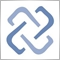"没有人可以信任, 除了自己"  (с)调试器

### 依据瞬时报价进行交易的理念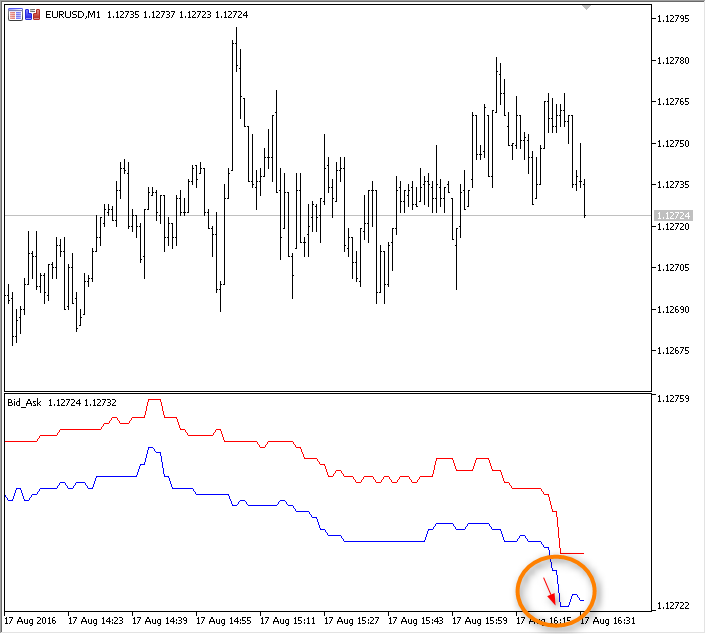`+1, 0, +2, -1, 0, +1, -2, -1, +1, -5, -1, +1, 0, -1, +1, 0, +2, -1, +1, +6, -1, +1,...`

### 创建瞬时报价指标

• ticks — 计算标准差时所用的瞬时报价数量
• gap — 获取标准差的间隔系数。```//+------------------------------------------------------------------+
//|                                              TickSpikeHunter.mq5 |
//|                                 版权所有 2016, MetaQuotes 软件公司|
//|                                             https://www.mql5.com |
//+------------------------------------------------------------------+
#property copyright "版权所有 2016, MetaQuotes 软件公司"
#property version   "1.00"
#property indicator_separate_window
#property indicator_buffers 3
#property indicator_plots   2
//--- 绘制瞬时报价
#property indicator_label1  "瞬时报价"
#property indicator_type1   DRAW_LINE
#property indicator_color1  clrGreen
#property indicator_style1  STYLE_SOLID
#property indicator_width1  1
//--- 绘制信号
#property indicator_label2  "信号"
#property indicator_type2   DRAW_COLOR_ARROW
#property indicator_color2  clrRed,clrBlue,C'0,0,0',C'0,0,0',C'0,0,0',C'0,0,0',C'0,0,0',C'0,0,0'
#property indicator_style2  STYLE_SOLID
#property indicator_width2  1
//--- 输入参数
input int      ticks=50;         // 计算所用瞬时报价数量
input double   gap=3.0;          // 通道宽度的标准差
//--- 指标缓存区
double         TickPriceBuffer[];
double         SignalBuffer[];
double         SignalColors[];
//--- 价格变化计数器
int ticks_counter;
//--- 第一次指标调用
bool first;
//+------------------------------------------------------------------+
//| 自定义指标初始化函数                                              |
//+------------------------------------------------------------------+
int OnInit()
{
//--- 指标缓存区映射
SetIndexBuffer(0,TickPriceBuffer,INDICATOR_DATA);
SetIndexBuffer(1,SignalBuffer,INDICATOR_DATA);
SetIndexBuffer(2,SignalColors,INDICATOR_COLOR_INDEX);
//--- 设置空值, 在绘图时应被忽略
PlotIndexSetDouble(0,PLOT_EMPTY_VALUE,0);
PlotIndexSetDouble(1,PLOT_EMPTY_VALUE,0);
//--- 信号将作为图标输出
PlotIndexSetInteger(1,PLOT_ARROW,159);
//--- 初始化全局变量
ticks_counter=0;
first=true;
//--- 程序初始化成功
return(INIT_SUCCEEDED);
}
//+------------------------------------------------------------------+
//| 自定义指标迭代函数                                                |
//+------------------------------------------------------------------+
int OnCalculate(const int rates_total,
const int prev_calculated,
const datetime &time[],
const double &open[],
const double &high[],
const double &low[],
const double &close[],
const long &tick_volume[],
const long &volume[],
{
//---

//--- 返回 prev_calculated 的数值用于下次调用
return(rates_total);
}
//+------------------------------------------------------------------+

```

```//+------------------------------------------------------------------+
//| 自定义指标迭代函数                                                |
//+------------------------------------------------------------------+
int OnCalculate(const int rates_total,
const int prev_calculated,
const datetime &time[],
const double &open[],
const double &high[],
const double &low[],
const double &close[],
const long &tick_volume[],
const long &volume[],
{
//--- 在首次调用期间将指标缓存区清零并标记为序列
if(first)
{
ZeroMemory(TickPriceBuffer);
ZeroMemory(SignalBuffer);
ZeroMemory(SignalColors);
//--- 序列数组向后定位, 在这种情况下最方便
ArraySetAsSeries(SignalBuffer,true);
ArraySetAsSeries(TickPriceBuffer,true);
ArraySetAsSeries(SignalColors,true);
first=false;
}
//--- 使用当前的收盘价作为价格
double lastprice=close[rates_total-1];
//--- 瞬时报价计数
ticks_counter++;
ApplyTick(lastprice); // 执行计算和缓存区移位
//--- 返回 prev_calculated 的数值用于下次调用
return(rates_total);
}
//+------------------------------------------------------------------+
//| 应用瞬时报价进行计算                                              |
//+------------------------------------------------------------------+
void ApplyTick(double price)
{
int size=ArraySize(TickPriceBuffer);
ArrayCopy(TickPriceBuffer,TickPriceBuffer,1,0,size-1);
ArrayCopy(SignalBuffer,SignalBuffer,1,0,size-1);
ArrayCopy(SignalColors,SignalColors,1,0,size-1);
//--- 保存最后的价格值
TickPriceBuffer=price;
//---
}

```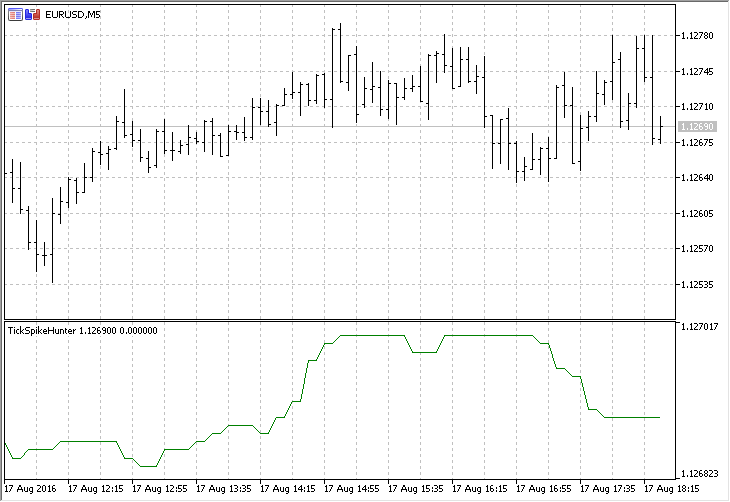```//--- 只在价格变化时计算
if(lastprice!=TickPriceBuffer)
{
ticks_counter++;      // 瞬时报价计数
ApplyTick(lastprice); // 执行计算和缓存区移位
}

```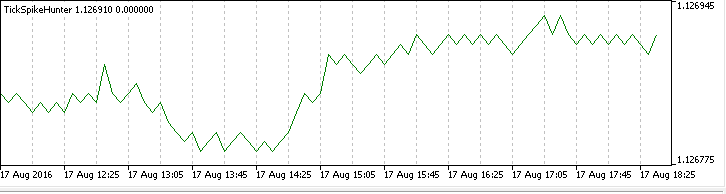### 添加一个辅助缓存区并计算标准差

```#property indicator_separate_window
#property indicator_buffers 4
#property indicator_plots   2
...
//--- 指标缓存区
double         TickPriceBuffer[];
double         SignalBuffer[];
double         DeltaTickBuffer[];
double         ColorsBuffers[];
...
//+------------------------------------------------------------------+
//| 自定义指标初始化函数                                              |
//+------------------------------------------------------------------+
int OnInit()
{
//--- 指标缓存区映射
SetIndexBuffer(0,TickPriceBuffer,INDICATOR_DATA);
SetIndexBuffer(1,SignalBuffer,INDICATOR_DATA);
SetIndexBuffer(2,SignalColors,INDICATOR_COLOR_INDEX);
SetIndexBuffer(3,DeltaTickBuffer,INDICATOR_CALCULATIONS);
...
}
//+------------------------------------------------------------------+
//| 自定义指标迭代函数                                                |
//+------------------------------------------------------------------+
int OnCalculate(const ...)

//--- 在首次调用期间将指标缓存区清零并标记为序列
if(first)
{
ZeroMemory(TickPriceBuffer);
ZeroMemory(SignalBuffer);
ZeroMemory(SignalColors);
ZeroMemory(DeltaTickBuffer);
//--- 序列数组向后定位, 在这种情况下最方便
ArraySetAsSeries(TickPriceBuffer,true);
ArraySetAsSeries(SignalBuffer,true);
ArraySetAsSeries(SignalColors,true);
ArraySetAsSeries(DeltaTickBuffer,true);
first=false;
}
...
return(rates_total);
}
//+------------------------------------------------------------------+
//| 应用瞬时报价进行计算                                              |
//+------------------------------------------------------------------+
void ApplyTick(double price)
{
int size=ArraySize(TickPriceBuffer);
ArrayCopy(TickPriceBuffer,TickPriceBuffer,1,0,size-1);
ArrayCopy(SignalBuffer,SignalBuffer,1,0,size-1);
ArrayCopy(SignalColors,SignalColors,1,0,size-1);
ArrayCopy(DeltaTickBuffer,DeltaTickBuffer,1,0,size-1);
//--- 保存最后的价格值
TickPriceBuffer=price;
//--- 计算与前一数值的差值
DeltaTickBuffer=TickPriceBuffer-TickPriceBuffer;
//--- 得到标准差
double stddev=getStdDev(ticks);

```

```//+------------------------------------------------------------------+
//| "暴力" 计算标准差                                                 |
//+------------------------------------------------------------------+
double getStdDev(int number)
{
double summ=0,sum2=0,average,stddev;
//--- 统计变化之和, 并计算期望收益
for(int i=0;i<ticks;i++)
summ+=DeltaTickBuffer[i];
average=summ/ticks;
//--- 现在计算标准差
sum2=0;
for(int i=0;i<ticks;i++)
sum2+=(DeltaTickBuffer[i]-average)*(DeltaTickBuffer[i]-average);
stddev=MathSqrt(sum2/(number-1));
return (stddev);
}

```

```//+------------------------------------------------------------------+
//| 应用瞬时报价进行计算                                              |
//+------------------------------------------------------------------+
void ApplyTick(double price)
{
int size=ArraySize(TickPriceBuffer);
ArrayCopy(TickPriceBuffer,TickPriceBuffer,1,0,size-1);
ArrayCopy(SignalBuffer,SignalBuffer,1,0,size-1);
ArrayCopy(SignalColors,SignalColors,1,0,size-1);
ArrayCopy(DeltaTickBuffer,DeltaTickBuffer,1,0,size-1);
//--- 保存最后的价格值
TickPriceBuffer=price;
//--- 计算与前一数值的差值
DeltaTickBuffer=TickPriceBuffer-TickPriceBuffer;
//--- 得到标准差
double stddev=getStdDev(ticks);
//--- 如果价格变化超出指定的阀值
if(MathAbs(DeltaTickBuffer)>gap*stddev) // 将会在第一个瞬时报价上显示一个信号, 保留它作为一个 "特征"
{
SignalBuffer=price;     // 放置一个圆点
string col="Red";          // 圆点省缺为红色
if(DeltaTickBuffer>0)   // 价格暴涨
{
SignalColors=1;      // 则圆点为蓝色
col="Blue";             // 保存日志
}
else                       // 价格暴跌
SignalColors=0;         // 圆点为红色
//--- 输出消息至智能程序日志
PrintFormat("瞬时报价=%G 变化=%.1f pts, 触发=%.3f pts,  标准差=%.3f pts %s",
TickPriceBuffer,DeltaTickBuffer/_Point,gap*stddev/_Point,stddev/_Point,col);
}
else SignalBuffer=0;       // 无信号
//---
}

```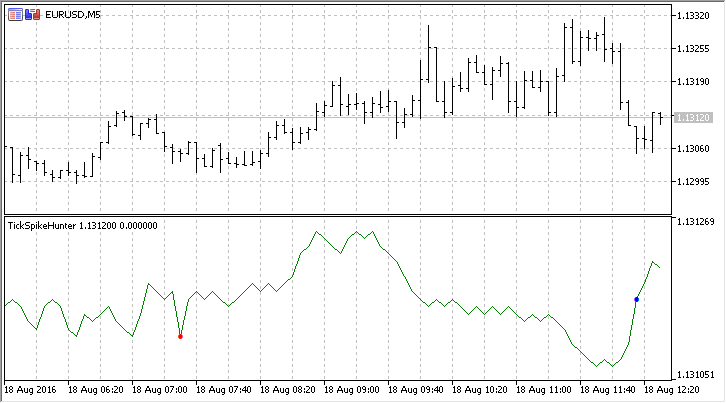### 代码剖析: 操作提速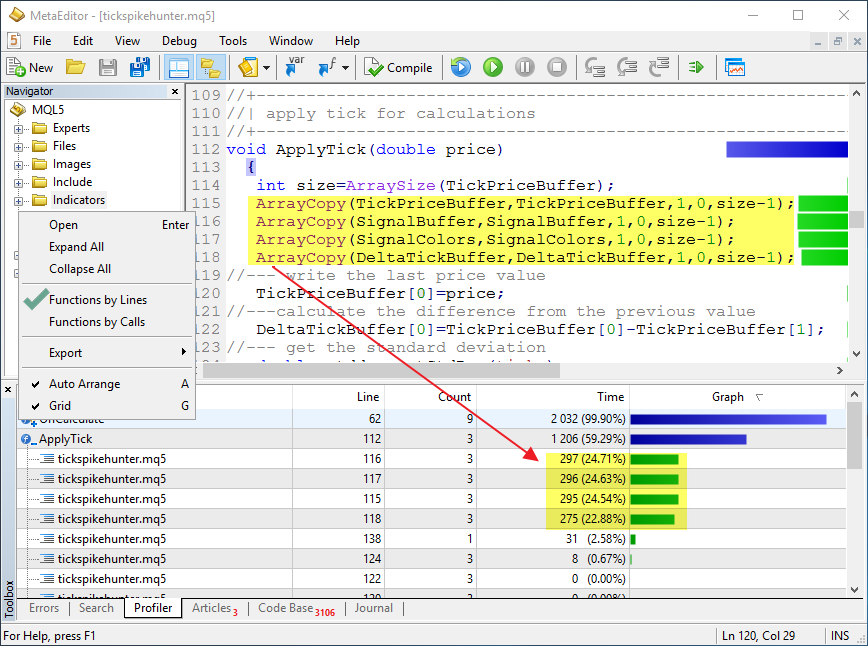```//--- 输入参数
input int      ticks=50;         // 计算所用瞬时报价数量
input int      shift=200;        // 数值移位的数量
input double   gap=3.0;          // 通道宽度的标准差
...
void ApplyTick(double price)
{
//--- 每笔瞬时报价时指标缓存区需要移位的元素数量
int move=ArraySize(TickPriceBuffer)-1;
if(shift!=0) move=shift;
ArrayCopy(TickPriceBuffer,TickPriceBuffer,1,0,move);
ArrayCopy(SignalBuffer,SignalBuffer,1,0,move);
ArrayCopy(SignalColors,SignalColors,1,0,move);
ArrayCopy(DeltaTickBuffer,DeltaTickBuffer,1,0,move);

```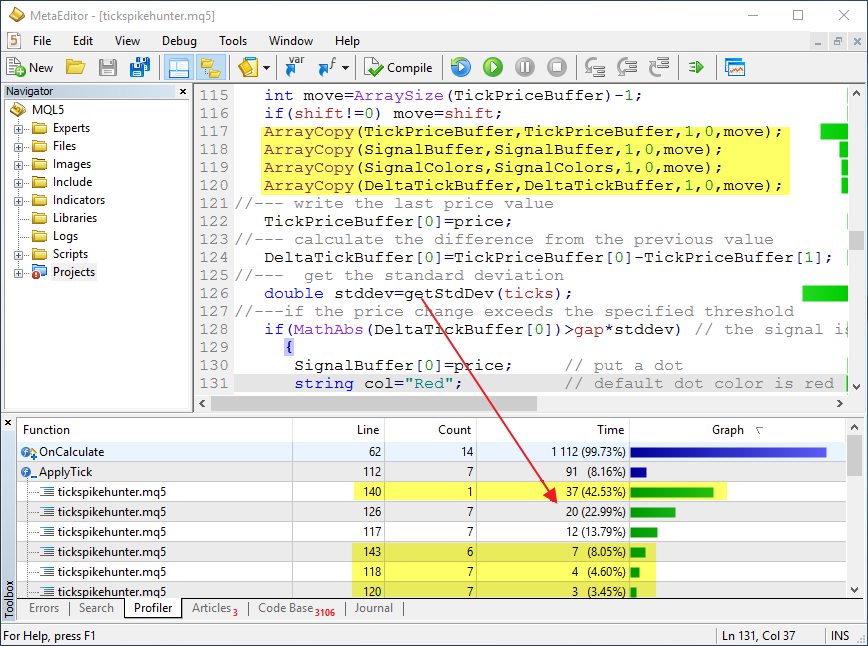### 分析代码优化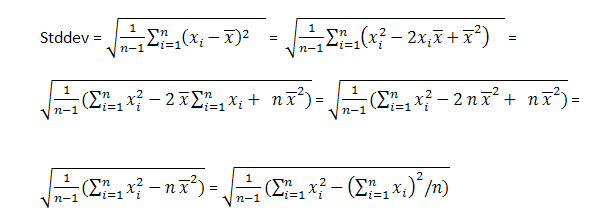```//+------------------------------------------------------------------+
//| 基于公式计算标准差                                                |
//+------------------------------------------------------------------+
double getStdDevOptimized(int number)
{
//---
static double X2[],X[],X2sum=0,Xsum=0;
static bool firstcall=true;
//--- 首次调用
if(firstcall)
{
//--- 设置动态数组大小, 大于瞬时报价数量加一
ArrayResize(X2,ticks+1);
ArrayResize(X,ticks+1);
//--- 在计算初始, 确保非零数值
ZeroMemory(X2);
ZeroMemory(X);

firstcall=false;
}
//--- 数组移位
ArrayCopy(X,X,1,0,ticks);
ArrayCopy(X2,X2,1,0,ticks);
//--- 计算新的接收数值合计
X=DeltaTickBuffer;
X2=DeltaTickBuffer*DeltaTickBuffer;
//--- 计算新合计
Xsum=Xsum+X-X[ticks];
X2sum=X2sum+X2-X2[ticks];
//--- 标准方差
double S2=(1.0/(ticks-1))*(X2sum-Xsum*Xsum/ticks);
//--- 统计瞬时报价之和, 并计算期望收益
double stddev=MathSqrt(S2);
//---
return (stddev);
}

```

```//--- 计算与前一数值的差值
DeltaTickBuffer=TickPriceBuffer-TickPriceBuffer;
//--- 得到标准差
double stddev=getStdDev(ticks);
double std_opt=getStdDevOptimized(ticks);

```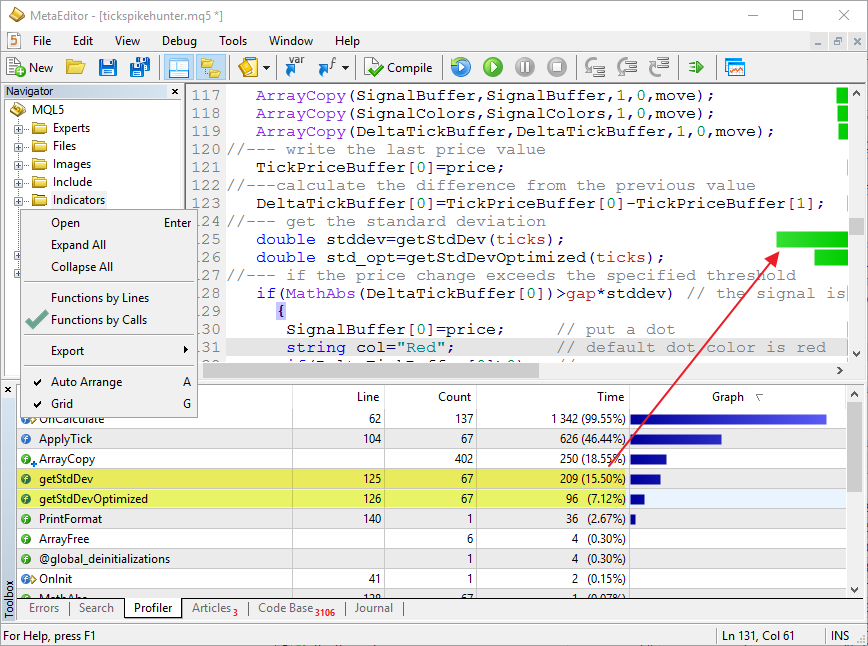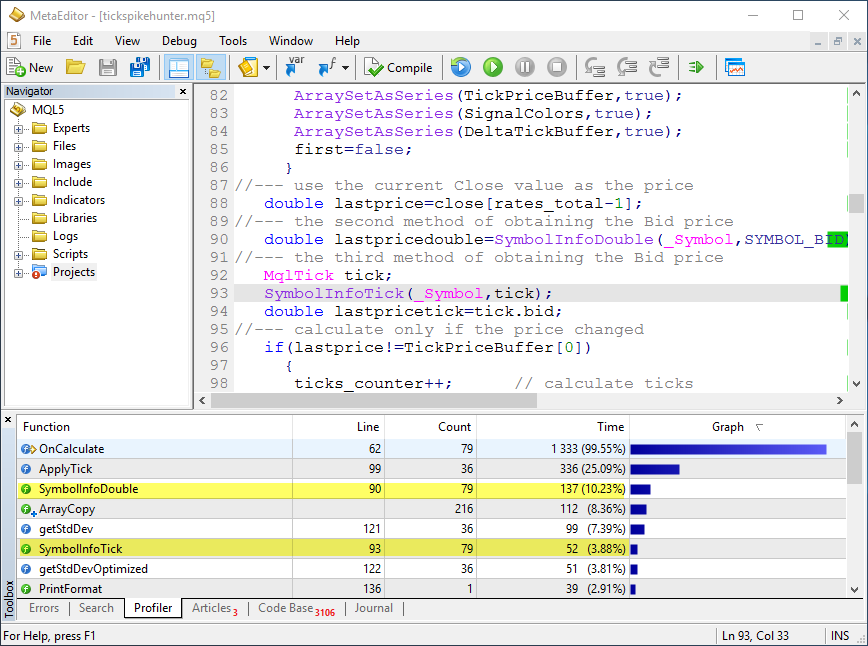### 在测试器里基于实际的瞬时报价调试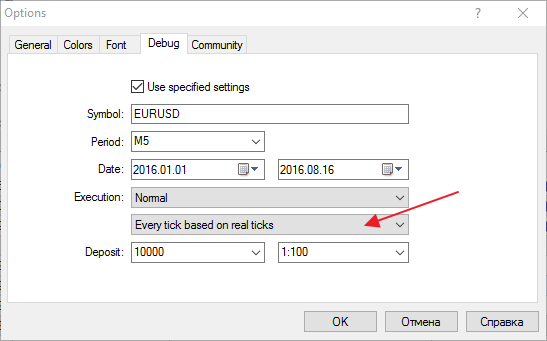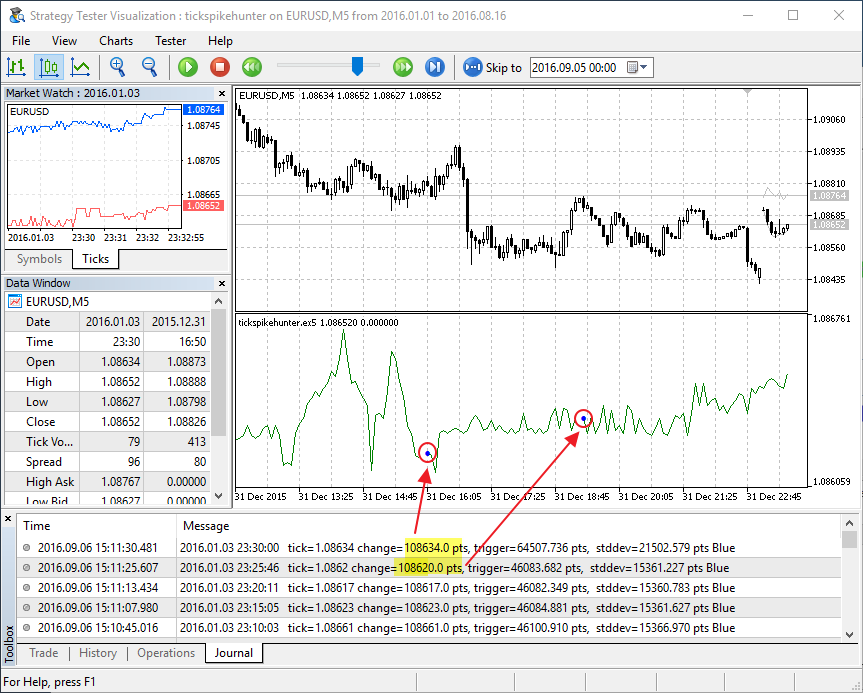```void ApplyTick(double price)
{
//--- 保存 TickPriceBuffer 数组大小 - 它等于图表上的柱线数量
static int prev_size=0;
int size=ArraySize(TickPriceBuffer);
//--- 如果指标缓存区的大小无变化, 向后移动元素 1 个位置
if(size==prev_size)
{
//--- 每笔瞬时报价时指标缓存区需要移位的元素数量
int move=ArraySize(TickPriceBuffer)-1;
if(shift!=0) move=shift;
ArrayCopy(TickPriceBuffer,TickPriceBuffer,1,0,move);
ArrayCopy(SignalBuffer,SignalBuffer,1,0,move);
ArrayCopy(SignalColors,SignalColors,1,0,move);
ArrayCopy(DeltaTickBuffer,DeltaTickBuffer,1,0,move);
}
prev_size=size;
//--- 保存最后的价格值
TickPriceBuffer=price;
//--- 计算与前一数值的差值

```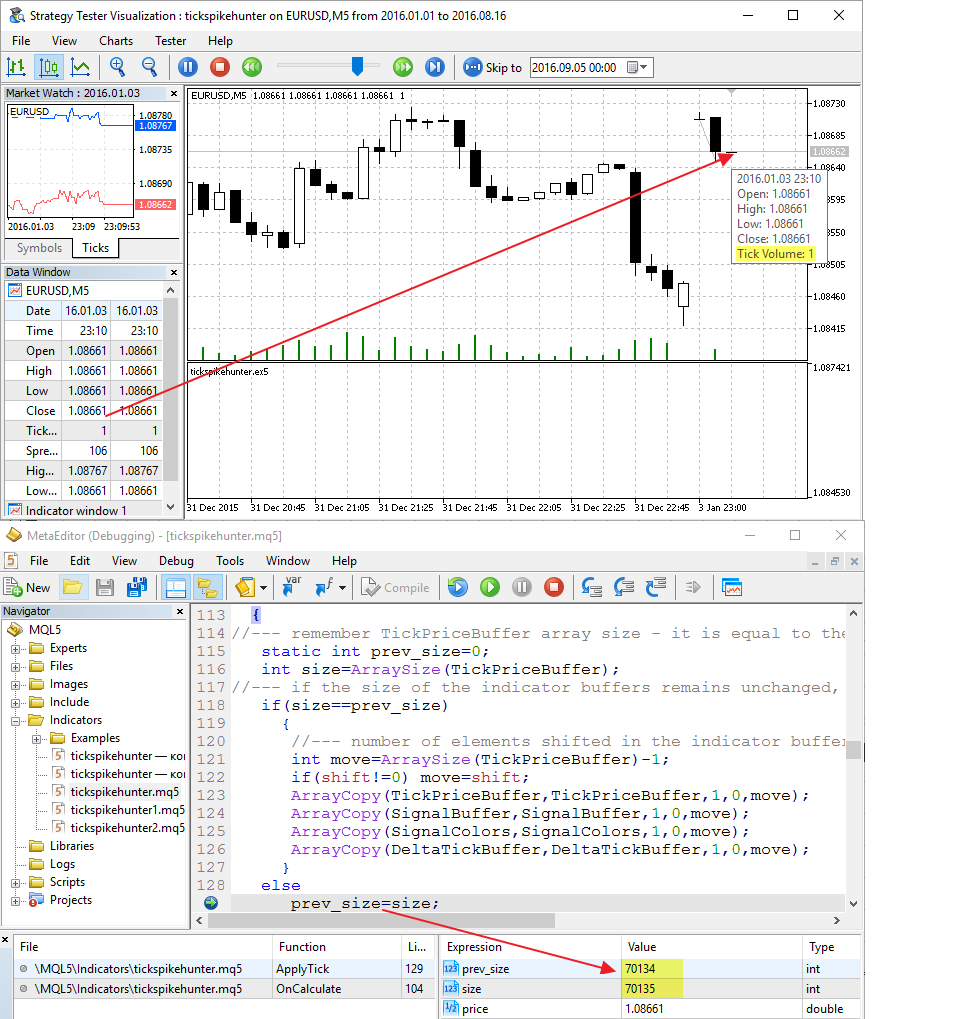### MetaEditor 是开发交易策略的一个现成实验室

1. 如何在一两分钟内创建瞬时报价图表;
2. 如何在图表上通过按 F5 键使用实时模式调试;
3. 如何运行代码剖析来确定低效的代码部分;
4. 如何在可视l测试模式里基于历史数据进行快速调试;
5. 如何在调试期间查看所需变量的数值。

#### 该作者的其他文章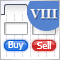图形界面 VIII: 日历控件 (第一章)MQL5 酷宝书 - 移动通道交易信号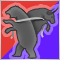Erik Nayman 的绳索指标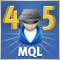跨平台智能交易程序: 重用来自 MQL5 标准库的控件